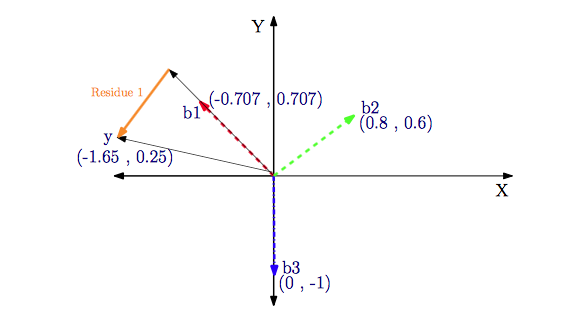# 图像的稀疏表征

n×n

y=Dx

minx||x||0,s.t.y=Dx

MP算法是稀疏表征中用于稀疏求解的最基本方法之一. 我在学习过程中参考网上一些资料，觉得大部分写得比较理论化，看起来稍微吃力一些. 阅读了Koredianto Usman的Introduction to Matching Pursuit(MP)一文，我觉得这篇文章写得很不错，从实例出发，很好接. 这篇博文是我对该文章翻译的基础上而写的.

1. 原文中有一些小错误，我在译文中进行了修改. 有对照原文阅读的同学，若发现有不一致，请不要奇怪.
2. 所有计算结果都保留两位小数.

# 问题提出

x=1.210

A=(0.7070.7070.80.601)

（注：原文中称 A $\mathrm{A}$为measurement matrix）

# 匹配追踪

b1=(0.7070.707)b2=(0.80.6)b3=(01)

Ax $\mathrm{A}\cdot x$是原子 b1 $b_1$ b2 $b_2$ b3 $b_3$的线性组合

Ax=(0.7070.7070.80.601)1.210=1.2(0.7070.707)+1(0.80.6)+0(01)=y=(1.650.25)

1. 选择对y$y$值贡献最大的原子 pi=maxj<bj,y> $p_i=\max_j$

• 计算差值 ri=ri1pi<ri1,pi> $r_i = r_{i-1} - p_i \cdot $ （注：该公式在原文中稍微有点问题，这里做了修正. 对于 r0=y $r_0=y$
• 选择剩余原子中与 ri $r_i$内积最大的
• 重复步骤2和3，直到差值小于给定的阈值（稀疏度）
• 下面进行实例计算：

首先，分别计算 y $y$b1$b_1$ b2 $b_2$ b3 $b_3$的内积：

<y,b1>=1.34,<y,b2>=1.17,<y,b3>=0.25
<script type="math/tex; mode=display" id="MathJax-Element-51"> =-1.34, \qquad =1.17, \qquad =0.25</script>

取绝对值以后，我们可以发现 b1 $b_1$ y $y$得到最大的内积值. 然后，在第一步中我们选择b1$b_1$. 接下来计算差值：

r1=yb1<y,bi>=(1.650.25)(1.34)(0.7070.707)=(0.700.70) $r_1 = y - b_1 \cdot = \begin{pmatrix}1.65 \\ -0.25\end{pmatrix} - (-1.34) \cdot \begin{pmatrix}-0.707 \\ 0.707\end{pmatrix} = \begin{pmatrix}0.70 \\ 0.70\end{pmatrix}$

接来下，计算差值和 b2 $b_2$ b3 $b_3$的内积：

<r1,b2>=0.98<r1,b3>=0.70
<script type="math/tex; mode=display" id="MathJax-Element-58"> =0.98 \qquad =-0.70</script>

取绝对值以后， b2 $b_2$ y $y$值的贡献最大。

接下来，计算差值r2=r1b2<r1,b2>=(0.70.7)(0.80.6)0.98=(0.080.11)$r_2 = r_1 - b_2 \cdot = \begin{pmatrix}0.7 \\ 0.7\end{pmatrix} - \begin{pmatrix}0.8 \\ 0.6\end{pmatrix} \cdot 0.98 = \begin{pmatrix}-0.08 \\ 0.11\end{pmatrix}$

最后，计算 r2 $r_2$ b3 $b_3$的内积： <r2,b3>=0.11 <script type="math/tex" id="MathJax-Element-64"> =-0.11</script>

所以，最后的三个稀疏稀疏是 1.340.980.11 $\begin{pmatrix}-1.34 \\ 0.98 \\ -0.11\end{pmatrix}$

这和准确的系数 1.210 $\begin{pmatrix}-1.2 \\ 1 \\ 0\end{pmatrix}$很接近

反酸回去，和给定的 y $y$也很接近.

Ax=(0.7070.7070.80.601)1.340.980.11=(1.730.25)

# MP算法实质

从下面的图，我们可以很清楚地看到MP算法的实质：就是利用原子向量的线性运算去逐渐去逼近信号向量，经过不停地迭代，最后达到给定的稀疏度.

匹配追踪算法可以直接得到信号稀疏性的表达. 以贪婪迭代的方法选择 D $\mathrm{D}$的列，使得在每次迭代的过程中所选择的列与当前冗余向量最大程度的相关.11-29
06-17
03-13
12-06
04-123万+
04-161397
04-1715万+
©️2020 CSDN 皮肤主题: 成长之路 设计师:Amelia_0503